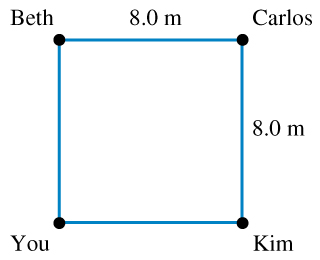# Problem: You and three friends stand at the corners of a square whose sides are 8.0 m long in the middle of the gym floor, as shown in the figure . You take your physics book and push it from one person to the other. The book has a mass of 1.6 kg , and the coefficient of kinetic friction between the book and the floor is k= 0.26.The book slides from you to Beth and then from Beth to Carlos, along the lines connecting these people. What is the work done by friction during this displacement?You slide the book from you to Carlos along the diagonal of the square. What is the work done by friction during this displacement?You slide the book to Kim who then slides it back to you. What is the total work done by friction during this motion of the book?Is the friction force on the book conservative or nonconservative?Explain.

###### FREE Expert Solution
82% (451 ratings)
###### Problem Details

You and three friends stand at the corners of a square whose sides are 8.0 m long in the middle of the gym floor, as shown in the figure. You take your physics book and push it from one person to the other. The book has a mass of 1.6 kg , and the coefficient of kinetic friction between the book and the floor is k= 0.26.

The book slides from you to Beth and then from Beth to Carlos, along the lines connecting these people. What is the work done by friction during this displacement?

You slide the book from you to Carlos along the diagonal of the square. What is the work done by friction during this displacement?

You slide the book to Kim who then slides it back to you. What is the total work done by friction during this motion of the book?

Is the friction force on the book conservative or nonconservative?

Explain.

Frequently Asked Questions

What scientific concept do you need to know in order to solve this problem?

Our tutors have indicated that to solve this problem you will need to apply the Intro to Calculating Work concept. You can view video lessons to learn Intro to Calculating Work. Or if you need more Intro to Calculating Work practice, you can also practice Intro to Calculating Work practice problems.